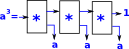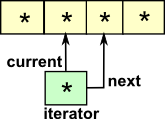# Data Type

Most programming have a set of built-in data types and then ways to combine them to produce more complex data types, for instance,

## Primitive Data Types

• Integer
• Float (real)
• Boolean (Logic)
• Character

There may also be some variations on these such as double precision.

The type definitions on this page use a programming language called 'Charity' (http://pll.cpsc.ucalgary.ca/charity1/www/home.html) which takes its ideas from category theory.

## Combining Primitive Data Types

We can combine the above built-in types to form compound types in the following ways:

### Union

This is where only one of the types applies at a given time.

We might want a type like Shape which could either be a Circle which requires a single float to define it (say radius) or alternatively a Rectangle which requires two floats to define it (say height and width).

type: Shape = Circle float | Rectangle float float

Another possibly might be where we require a function to normally return a numeric value but in case of an error, such as divide by zero, we want it to return a string containing an error message.

A similar concept is enumeration (enum)

### Compound (Tuple)Alternatively the compound type can contain both the data types being combined simultaneously. This is a bit like a fixed length list but where each element in the list could be a different type.

This page looks at data types as mathematical expressions which we can manipulate in equations.

## Recursive Types

We can define compound types recursively. There are two ways to do this:

• Inductive data types, such as list, where the recursion is on the input and the instance on the output of the definition.
• Coinductive data types, such as stream, where the recursion is on the output and the instance on the input of the definition.
inductive coinductive
example type List A = cons:(A,List A)->* | nil:()->* type Stream A = first:*->(A) & rest:*->(Stream A)
definition An inductively defined set is the least solution defined by some relation (inequality). If some other set obeys the relation then it contains the defined set. A coinductivly defined set is the greatest solution defined by some relation (inequality). If some other set obeys the relation then the defined set contains the coinductivly defined set.

## Algebraic Data Types

Languages such as Haskell and Idris allow types to be defined by listing their possible values, these are the constructors for the type.

These constructors can also be recursive allowing us to define infinite types such as natural numbers and lists.

Types defined in this way allow us to prove things about the type at compile time, especially if the type is defined as a free initial algebra such as natural numbers defined in terms of zero and successor constructors. We can then define plus in a way that we can prove what would otherwise be axioms.

Certain types, such as natural numbers and lists, are fully specified by these constructors. These are known as w-types [ref 1] . However more complex data types such as groups or graphs would be hard or impossible to fully define in this way although we can still construct something with the required functionality. For example, imagine we want to model a finite group and to do this we want to construct permutations. We could represent a permutation as a list of natural numbers where the value of the n-th item in the list represents the index of the entry it maps to. To be a valid permutation the list should contain every index once only but this is not enforced by the constructor.

 Perhaps one way to specify this would be to add equations to specify the conditions as in this psudo code: ```data perm Nat p : list Nat | p * (inv p) = 1```

This sort of thing can be done in proof assistants but I don't know any general purpose languages with this ability.

## Examples of Data Types#### List Data Type

This is inductive:
Construction is simpler but readout (destruction) is more complicated.#### Stream Data Type

This is coinductive:
Construction is more complicated but readout (destruction) is simpler.

## Object Oriented

In an object oriented program recursion can be used as follows:

### Java - List

In java we encapsulate in an object

```class List<E>
{
E value;
List<E> next;
}
```

### Scala - Tree

case classes in scala are a halfway between encapsulating in an object and algebraic data types in functional languages.

```abstract class Tree
case class Sum(l: Tree, r: Tree) extends Tree
case class Var(n: String) extends Tree
case class Const(v: Int) extends Tree```

The case class is similar to | in that it uses pattern matching.

## Charity

terminology

 1: X -> X identity !: Z -> 1 terminal map : W -> X * Y product factorisor P0: X * Y -> X first projection P1: X * Y -> Y second projection C = P0,P1 : X * Y = Y * X product symmetry Δ = <1,1>: X -> X * X product diagonal map : X + Y -> W coproduct factorisor b0: X -> X + Y first coprojection b1: Y -> X + Y second coprojection

where:

• x = W -> X
• y = W -> Y
• v = X -> W
• w = Y -> W

functor/mapping/function examples:

 nil: 1 -> C nil is a function which takes no inputs and returns a single value ss: A -> C ss is a function which takesone input and returns a single value cons: A * C -> C cons is a function which takes two inputs and returns a single value

#### utilities:

 list (built in) ```data list A -> C = nil : 1 -> C | cons: A * C -> C.``` the "success or failure" datatype: the datatype of exceptions ```data SF A -> C = ss: A -> C | ff: 1 -> C.``` the coproduct (sum) datatype ```data coprod(A, B) -> C = b0: A -> C | b1: B -> C.``` the boolean datatype (builtin) `data bool -> C = false | true: 1 -> C.` the natural number datatype ```data nat -> C = zero: 1 -> C | succ: C -> C.```

#### Inductive Datatypes

 bTree - binary tree ```data bTree (A, B) -> C = leaf: A -> C | node: B * (C * C) -> C.```

#### Coinductive Datatypes

 triple ```data T -> triple(A, B, C) = p0_3: T -> A | p1_3: T -> B | p2_3: T -> C.``` conat `data C -> conat = denat: C -> SF(C).` colist `data C -> colist(A) = delist: C -> SF(A * C).` cobTree `data C -> cobTree(A, B) = debTree: C -> A + (B * (C * C)).`

#### Infinite Datatypes

 infnat `data C -> infnat = poke: C -> C.` inflist ```data I -> inflist(A) = head: I -> A | tail: I -> I.``` infbTree `data C -> infbTree(B) = infnode: C -> B * (C * C).`

#### Lazy Datatypes

 Lid ```data Eid A -> C = eid: A -> C. % eager identify datatype data C -> Lid A = lid: C -> A. % lazy identify datatype``` Lprod ```data C -> Lprod(A, B) = fst: C -> A | snd: C -> B.``` Lnat ```data Lnat -> N = Lzero: 1 -> N | Lsucc: Lid(N) -> N.``` Llist ```data Llist(A) -> L = Lnil : 1 -> L | Lcons: A * Lid(L) -> L.```

#### Higher-Order Datatype

 exp ```The exponetial datatype: the datatype of total functions data C -> exp(A, B) = fn: C -> A => B.``` proc ```* the datatype of total, deteministic processes data C -> proc(A, B) = pr: C -> A => B * C. * the datatype of partial processes data C -> Pproc(A, B) = Ppr: C -> A => SF(B * C). * the datatype of nondeteministic processes data C -> NDproc(A, B) = NDpr: C -> A => list(B * C).``` stack ```data S -> stack(A) = push: S -> SF(A) => S | pop : S -> S * SF(A).``` queue ```data Q -> queue(A) = enq: Q -> SF(A) => Q | deq: Q -> Q * SF(A).```

[ref 1]

Advanced Topics in Programming Languages Series: Parametric Polymorphism and the Girard-Reynolds Isomorphism. This talk is based on a series of papers by Philip Wadler, a principal designer of the Haskell programming language. Featured are a number of double-barreled names in computer science:

• Hindley-Milner (Strong typing without having to type the types)
• Curry-Howard (Isomorphism between types and theorems, terms and proofs)
• Girard-Reynolds (Isomorphism between types and terms in the presence of parametricity)

For Djinn code see here.

Parametricity

Where I can, I have put links to Amazon for books that are relevant to the subject, click on the appropriate country flag to get more details of the book or to buy it from them.Computation and Reasoning - This book is about type theory. Although it is very technical it is aimed at computer scientists, so it has more discussion than a book aimed at pure mathematicians. It is especially useful for the coverage of dependant types.

Commercial Software Shop

Where I can, I have put links to Amazon for commercial software, not directly related to this site, but related to the subject being discussed, click on the appropriate country flag to get more details of the software or to buy it from them.

This site may have errors. Don't use for critical systems.